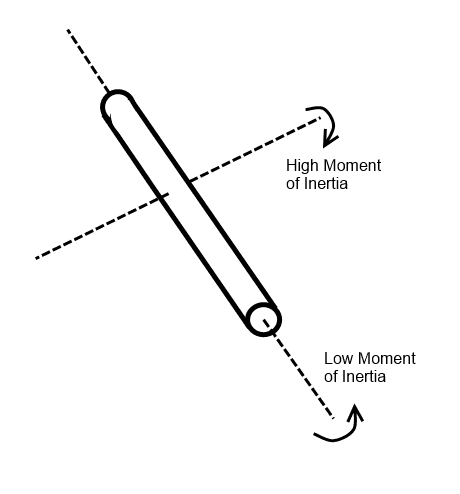# Is angular momentum position specific?

• avito009

#### avito009

Do we find out angular momentum for an object from a specific position? Since L= r x mv. R is the distance from the centre of mass. So can I say that angular momentum is 12 for a fixed position 4 metres away from centre of mass having mass of 1 kg and velocity of 3 metres per second north?

You mean the center of mass has a velocity of 3m/s?
If not, what has this velocity?

No not the centre of mass but the point 4 metres away from centre of mass.

So your "fixed position 4 m away from the center of mass" is actually moving with 3m/s?

Maybe you can describe your system. Is it a rigid body, a set of several bodies (and what kind of bodies), etc.

So your "fixed position 4 m away from the center of mass" is actually moving with 3m/s?

Maybe you can describe your system. Is it a rigid body, a set of several bodies (and what kind of bodies), etc.

It is a rigid body.

The angular momentum of the rigid body about a fixed point ##O## is calculated by the formula
##\boldsymbol L_O= m\boldsymbol{OS}\times \boldsymbol v_S+J_S\boldsymbol\omega##, here ##S## is the center of mass, ##m## is the mass of the rigid body, ##\boldsymbol v_S## is the velocity of the center of mass, ##J_S## is the operator of inertia about the center of mass, ##\boldsymbol\omega## is the angular velocity

Then what is moving with 3m/s?

Do we find out angular momentum for an object from a specific position? Since L= r x mv. R is the distance from the centre of mass. So can I say that angular momentum is 12 for a fixed position 4 metres away from centre of mass having mass of 1 kg and velocity of 3 metres per second north?
Angular momentum is calculated around a given point, and the value you calculate may be different for different points.

Angular momentum is calculated around a given point, and the value you calculate may be different for different points.

That answers one part of the question. But nasu confused me. So can you tell me that the velocity is the velocity of centre of mass or of the particular point? I mean what is v in the formula L=r x mv?

The formula ##\mathbf{L}=\mathbf{r} \times m\mathbf{v}## is really for a point mass, so there is only one velocity.
But there is a similar formula for a rigid body
##\mathbf{L} = \mathbf{R}_{cm} \times M\mathbf{V}_{cm} + \overleftrightarrow{I}\mathbf{\omega}##
The first term is the orbital angular momentum, measured using the velocity of the center of mass, and the second term is the spin angular momentum (nothing to do with the quantum mechanics term). Basically, if it's not spinning, you can just treat the center of mass as a point particle.

[Post merged with this thread by moderator]

L= R x MV Now what is R in this formula. At some places it is mentioned as radius and at others the distance from centre of mass. So what is R? Is it radius or distance from centre of mass?

Last edited by a moderator:
I note you have a few other threads running on a similar subject...

https://www.physicsforums.com/threa...onentum-mean-faster-spin.874282/#post-5490162

Perhaps it helps to understand that an objects moment of inertia can depend on which axis it is being rotated about. For example a long thin rod will have a low moment of inertia when rotated about an axis that goes down the centre of the rod and a high moment of inertial when rotated about an axis through the middle of the rod. This is because mass further from the axis increases the moment of inertia more than mass close to the axis (think leverage).I suggest reading up on how the moment if inertia is calculated for objects of different shapes. Basically you have to break the object down into small parts and then sum (integrate) the moment of inertia of all the component parts, each of which might be at a different radius from the axis of rotation.

If the object is rigid then all parts are rotating at the same angular velocity so when working out the angular momentum it doesn't matter if you do...

a) Calculate the angular momentum of it's component parts and add them up
or
b) Calculate the moment of inertia of it's component parts, add them up, and then multiply by the angular velocity.